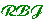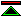Threads in the Web of Mathematics

These threads link the past, present and future of selected aspects of mathematics, helping to anchor speculation about the future in knowledge of the past.

 Rigour Rigour is about how carefully mathematical truths are checked before they are generally accepted as true. At any time mathematics will be conducted with varying standards of rigour, even by the best mathematicians (Ramanujan's work, over two thousand years after the Greeks established the need for proof, was highly valued even though results were not proven). Foundations Related to rigour is the idea of a foundation for mathematics, i.e. the idea that some branches of mathematics provide a basis on which other branches may be built. Continuity A particular problem zone which interacts heavily with the problem of rigour and the question of foundation is that of continuity. Since the beginnings of the science of mathematics the continuity of geometric lines has proven difficult to understand, and the relation between continuous and discrete quantities has been a source of paradox. Only with quite recent (historically) advances has it been possible to deal with these issues in a coherent manner, and there still remain gaps in the way this more coherent theoretical framework is applied in computational practice. Concepts Advances in mathematics are dependent on getting the right conceptual framework. Notation The utility of mathematical advances can be greatly abetted or mitigated by the felicity of the notations involved. This applies to notations conduction computations (numerals), notations for communication between humans and notations for communicating with computers. Computation Computation is a major feature of the application of mathematics. Computational techniques sometimes outstrip the explanatory powers of the theory and sometimes lag behind the theory. (see computing with real numbers) Mechanisation Mechanisation has only in recent times (the last couple of centuries) been a significant factor in doing mathematics. To the present day mechanised mathematics remains a pale imitation of the mathematical capabilities of human mathematicians. This thread lies more in the future than the past. Application It is the applications of mathematics which generate the economic imperatives. It is the applications of mechanised mathematics which will determine the pace and direction of mechanisation.©created 96/8/5 modified 96/10/5